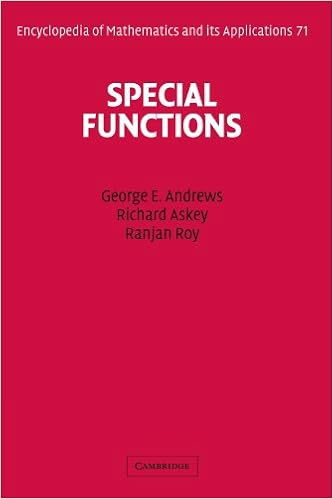## Wednesday, July 29, 2015

### Special Functions (Encyclopedia of Mathematics and its Applications) by George E. Andrews, Richard Askey, Ranjan RoySpecial functions, which include the trigonometric functions, have been used for centuries. Their role in the solution of differential equations was exploited by Newton and Leibniz, and the subject of special functions has been in continuous development ever since. In just the past thirty years several new special functions and applications have been discovered. This treatise presents an overview of the area of special functions, focusing primarily on the hypergeometric functions and the associated hypergeometric series. It includes both important historical results and recent developments and shows how these arise from several areas of mathematics and mathematical physics. Particular emphasis is placed on formulas that can be used in computation. The book begins with a thorough treatment of the gamma and beta functions that are essential to understanding hypergeometric functions. Later chapters discuss Bessel functions, orthogonal polynomials and transformations, the Selberg integral and its applications, spherical harmonics, q-series, partitions, and Bailey chains. This clear, authoritative work will be a lasting reference for students and researchers in number theory, algebra, combinatorics, differential equations, applied mathematics, mathematical computing, and mathematical physics.

Download
Download